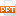# Chapter 2 Pattern Representation

The Cayley-Hamilton Theorem In practical calculation, we set the characteristic polynomial equal to zero, given the characteristic equation: det |A - I| = 0 The zeros of the characteristic polynomial, which are solutions to this equation, are the eigenvalues of the matrix A. The second step in solving the eigenvalue problem is to find the eigen vectors that correspond to each eigenvalue found in the solution of the characteristic equation.76 trang | Chia sẻ: vutrong32 | Ngày: 17/10/2018 | Lượt xem: 331 | Lượt tải: 0
Bạn đang xem trước 20 trang tài liệu Chapter 2 Pattern Representation, để xem tài liệu hoàn chỉnh bạn click vào nút DOWNLOAD ở trên
•chapter_2_8768.ppt